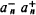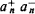# Commutation Relations

## Commutation Relations

fundamental relations in quantum mechanics that establish the connection between successive operations on the wave function, or state vector, of two operators (1 and L̂2) in opposite orders, that is, between 12 and 21. The commutation relations define the algebra of the operators. If the two operators commute, that is, 12 = L̂21, then the corresponding physical quantities L1 and L2 can have simultaneously defined values. But if their commutator is nonzero, that is, L̂L̂12 – L̂21 = c, then the uncertainty principle holds between the corresponding physical quantities: ΔL̂1ΔL̂2 ≤ ǀcǀ/2, where ΔL1 and ΔL2 are the uncertainties, or dispersions, of the measured values of the physical quantities L1 and L2. The commutation relation between the operator of the generalized coordinate and its conjugate generalized momentum (q̂p̂p̂ĝ = , where ħ is Planck’s constant) is very important in quantum mechanics. If the operator commutes with the operator of the total energy of the system (the Hamiltonian) , that is, L̂Ĥ = ĤL̂, then the physical quantity L (its average value, dispersion, and so on) preserves its value in time.

The commutation relations for the operators of the creation a+ and annihilation a of particles are of fundamental importance in the quantum mechanics of systems of identical particles and in quantum field theory. For a system of free (noninteracting) bosons, the particle creation operator in the state n,and the annihilation operator for the same particle,, satisfy the commutation relationfor fermions, the relationholds. The latter commutation relation is a formal expression of the Pauli principle.

V. B. BERESTETSKII

References in periodicals archive ?
Commutation Relations, Normal Ordering, and Stirling Numbers
They cover the structure of the space of the physical states, inequivalent representations of the canonical commutation relations, the spontaneous breakdown of symmetry and the Goldstone theorem, the dynamical rearrangement of symmetry and macroscopic manifestations of quantum field theory, thermal field theory and trajectories in the space of the representations, selected topics in thermal field theory, topological defects as non-homogeneous condensates, and dissipation and quantization.
In this respect, the vector-operators [MATHEMATICAL EXPRESSION NOT REPRODUCIBLE IN ASCII] satisfy the Bose commutation relations as:
Application of (12), (21) and (13), (22) to (14) and (15), and taking the Bose commutation relations presented above, we obtain
n] satisfying Poisson brackets or as a quantum system with the generators of the Lie algebra sl (2) and commutation relations
p+1,q] and found simple commutation relations in [Cl.
It is then straightforward to verify that the above commutation relations are
The articles include Karasev's "Noncommutative Algebras, Nanostructures, and Quantum Dynamics Generated by Resonances," Karasev and Novikova's "Algebras with Polynomial Commutation Relations for a Quantum Particle in Electric and Magnetic Fields," Vorobjjev's "Poisson Structures and Linear Euler Systems over Symplectic Manifolds," and also his "Poisson Equivalence over a Symplectic Leaf.
The first is the canonical commutation relations of the infinite-dimensional Heisenberg algebra, or oscillator algebra.
The idea is to reduce the conditions we get from the Jacobi identities to some well-known commutation relations.
The last relations are the well-known commutation relations of the Lorentz group generators.
We assume that the creation and annihilation operators of universes obey the standard commutation relations

Site: Follow: Share:
Open / Close